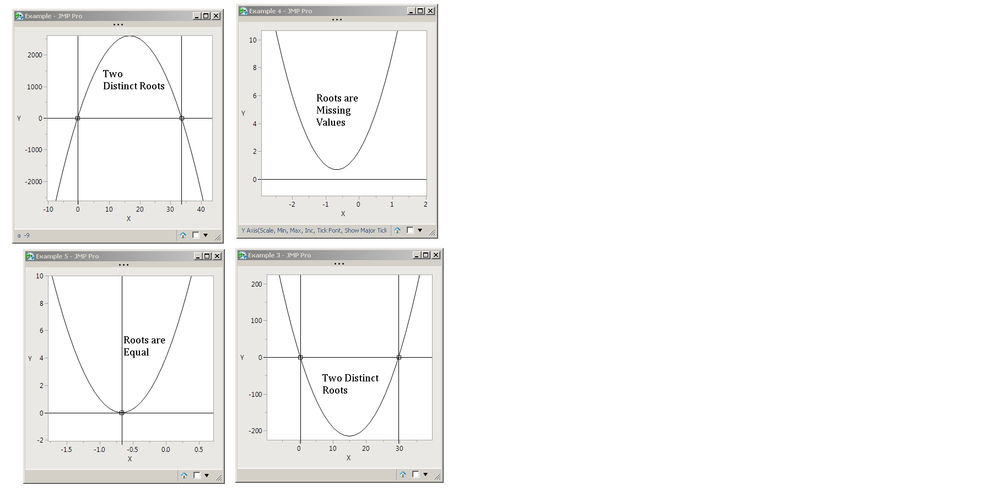Choose Language Hide Translation Bar
Highlighted

## roots of degree 2 polynomial

Hello everyone,

Does anyone know how to calculate roots of degree 2 polynomial with JMP scripting ?

I have the coefficients of the equation :  0 = A + B.X + C.X² . What I need is the real solutions.

I started to create a new data table with lots of rows in order to get as close as possible to the desired value, but it takes too much time.

Thanks,

3 REPLIES 3
Highlighted

## Re: roots of degree 2 polynomial

Isn't it the quadratic formula? In JSL:

``````NamesDefaultToHere(1);

// Usual to write a qyadratic as 'a*x^2 + b*x + c'...
a = 1; b = -30; c = 10;
root1 = (-b + sqrt(b^2 - 4*a*c))/(2*a);
root2 = (-b - sqrt(b^2 - 4*a*c))/(2*a);
Print(Char(Round(root1, 5))||" "||Char(Round(root2, 5)));
Print(Char(Round(a*root1^2 + b*root1 + c, 5))||" "||Char(Round(a*root2^2 + b*root2 + c, 5)));``````

If the roots are complex, the calculated roots are returned as '.'.

Highlighted

## Re: roots of degree 2 polynomial

needs parens around 2*a. Here it is with a graph; the slope of the line is zero at the minimum or maximum value that helps plan the axes for the graphs.

``````//a = -9; b = 300; c = 100; // opens downward
//a = 9; b = 12; c = 4; // single root
a = 2; b = -30; c = 10; // opens upward
//a = 3; b = 4; c = 2; // missing roots

root1 = (-b + Sqrt( b ^ 2 - 4 * a * c )) / (2 * a);
root2 = (-b - Sqrt( b ^ 2 - 4 * a * c )) / (2 * a);
Print( Char( Round( root1, 5 ) ) || " " || Char( Round( root2, 5 ) ) );
Print( Char( Round( a * root1 ^ 2 + b * root1 + c, 5 ) ) || " " || Char( Round( a * root2 ^ 2 + b * root2 + c, 5 ) ) );

// derivative(a*x^2+b*x+c,x) == 2 * x * a + b
center = -b / (2 * a); // slope is zero here
vcenter = a * center ^ 2 + b * center + c;

x1 = If( Is Missing( root1 ),
center,
root1
);
x2 = If( Is Missing( root2 ),
center,
root2
);

New Window( "Example",
Graph Box(
Frame Size( 300, 300 ),
X Scale( Min( x1, x2 ) - 10, Max( x1, x2 ) + 10 ),
Y Scale( -Abs( vcenter ) - 10, Abs( vcenter ) + 10 ),
<<yaxis( {Add Ref Line( 0, "Solid", "Black", "", 1 )} ),
<<xaxis( {Add Ref Line( root1, "Solid", "Black", "", 1 )} ),
<<xaxis( {Add Ref Line( root2, "Solid", "Black", "", 1 )} ),
Marker( Marker State( 3 ), {root1, 0}, {root2, 0} );
Y Function( a * x ^ 2 + b * x + c, x );
)
);``````Several possibilities

Craige
Highlighted

## Re: roots of degree 2 polynomial

Ooops! I edited the post . . .

Article Labels

There are no labels assigned to this post.